Maths
Study Material

# Geometric Progression Problems with Solutions

Learn about Geometric Progression through solving examples. If stuck, check out the solutions under them.

5 minutes long
Posted by Tanwir Silar, 4/8/2021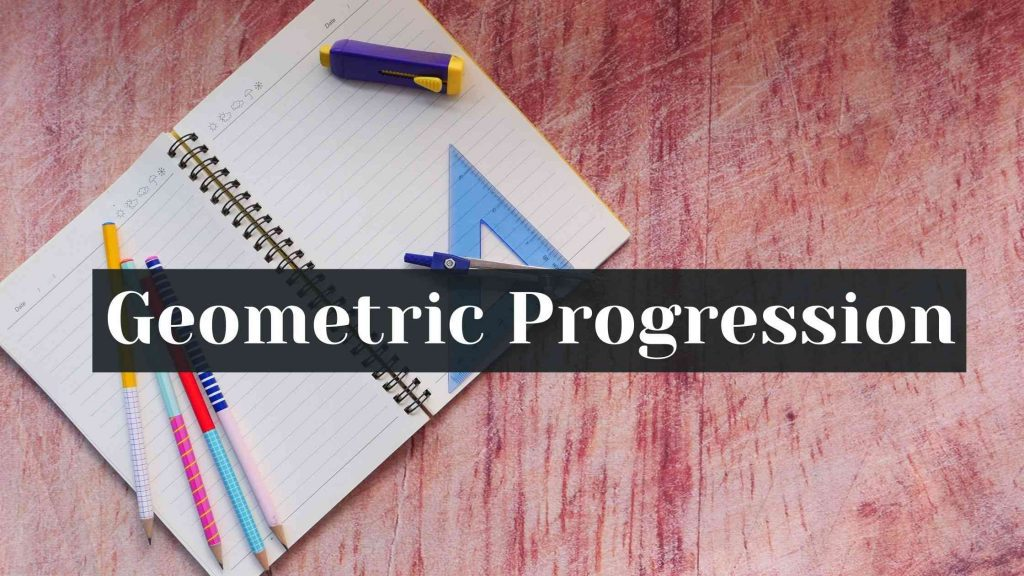Hesap Oluştur

Got stuck on homework? Get your step-by-step solutions from real tutors in minutes! 24/7. Unlimited.

Read here to learn about Geometric progression. In this blog, we are going to learn few approaches of solving Geometric Progression problems with solutions.

## Solved problems on Geometric Progression

Example 1:
Find the 20th and nth terms of the G.P.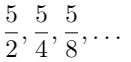Solution:
We have the first two terms of the G.P: a1 = 5/2, a2 = 5/4.
Common ratio of the G.P, r = an/an-1 = a2/a1 =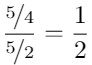Formula for the nth term of G.P. is, an = arn-1, where a = first term, r = common ratio.
Therefore, 20th term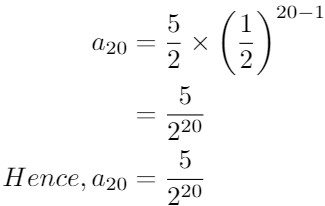Example 2:
Evaluate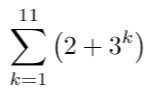Solution:
The given summations can be written as two summations.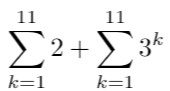The first summations adds the number 2 eleven times. So, the value of the summation = 11 x 2 = 22.
The second summation can be expanded as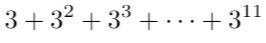This is a G.P. with first term, a = 3 and r = a2/a1 = 9/3 = 3.
We need to find the sum of first 11 terms of it. The formula for the sum of first n terms of a G.P. is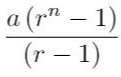Substituting the values of a, r, n in it, gives us :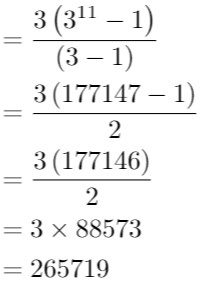The result of the second summations is 265719.
Answer = first summation + second summation
= 22 + 265719

#### More Examples:

Example 3:
The sum of first three terms of a G.P. is 39/10 and their product is 1. Find the common ratios and the terms.
Solution:
Note: When we are given the product of odd number (2n + 1) of terms of a G.P. It is preferred to take the sequence as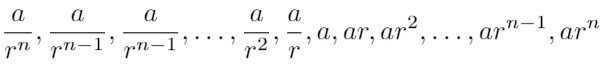. By taking the terms as stated when the product of all of the terms is taken, we are left with a2n+1, which reduces our effort significantly.
In the questions, we are given first three terms of G.P. So we consider the terms as.
We know that the sum of the terms is 39/10 and the product of the terms is 1, ⇒ a3 = 1 ⇒ a = 1. (real solution)
Now solving for using the equation of sum, we get: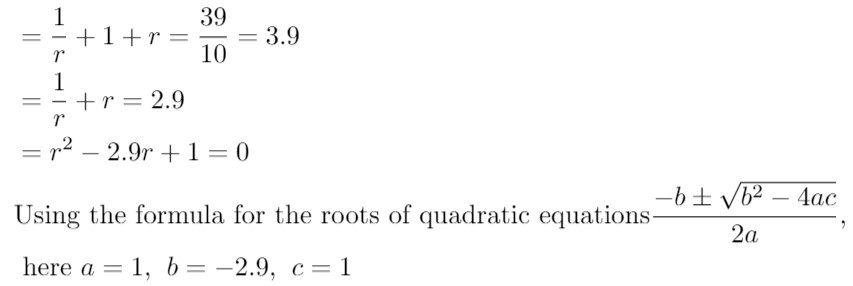Substituting the values in the expression we get the solutions as r = 0.4 or r = 2.5.
For r = 0.4 and a = 1, the terms of the G.P. are 1/0.4, 1, 0.4 = 2.5, 1, 0.4 .
For r = 2.5 and a = 1, the terms of the G.P. are 1/2.5, 1, 2.5 = 0.4, 1, 2.5 .

The above three approaches can be used to get solutions for a ton of problems on Geometric Progression.